Numerical Problems(Solved): Force and Newton's Law of Motion

# Numerical Problems(Solved): Force and Newton's Law of Motion - Science Class 9

Ex.1 Calculate the force required to impact to a car, a velocity of 30 m s-1 in 10 seconds. The mass of the car is 1,500 kg.
Sol. Here u = 0 m s-1; v = 30 ms-1; t = 10 s; a = ?
Using v = u +at, we have
30 = 0+ a (10)
a = 3 m s-2
Now F = ma = 1,500 × 3
or F = 4,500 N.

Ex.2 A cricket ball of mass 70 g moving with a velocity of 0.5 m s-1 is stopped by player in 0.5 s. What is the force applied by player to stop the ball?
Sol. Here m = 70 g = 0.070 kg; u = 0.5 m s-1; v = 0; t = 0.5 s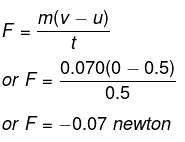Ex.3 What will be acceleration of a body of mass 5 kg if a force of 200 N is applied to it?
Sol. Here m = 5 kg; F = 200 N
F = ma or a = F/m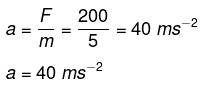Ex.4 A bullet of mass 10 g is fired from a rifle. The bullet takes 0.003 s to move through its barrel and leaves with a velocity of 300 ms-1. What is the force exerted on the bullet by the rifle?
Sol. Here m = 10 g = 0.010 kg ; u = a ; v = 300 m s-1
t = 0.003 s, F = ?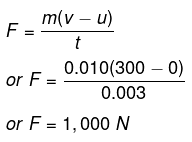Ex.5 What force would be needed to produce an acceleration of 1 ms-2 on a ball of mass 1 kg?
Sol. Here m = 1 kg; a = 1 ms-2 ; F = ?
Now F = m a = 1 × 1
or F = 1 newton.

Ex.6 What is the acceleration produced by a force of 5 N exerted on an object of mass 10 kg?
Sol. Here F = 5 N; m = 10 kg; a = ?
Now F = ma o
a = 0.5 ms-2

Ex.7 How long should a force of 100 N act on a body of 20 kg so that it acquires a velocity of 100 ms-1
Sol. Here v _ u = 100 m s-1, m = 20 kg; F = 100 N ;t = ?
We know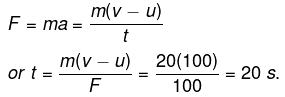Ex.8 A 1,000 kg vehicle moving with a speed of 20 m s-1 is brought to rest in a distance of 50 m,
(i) Find the acceleration;
(ii) Calculate the unbalanced force acting on the vehicle;
(iii) The actual force applied by the brakes may be slightly less than that calculated in (ii). Why? Give reason.

Sol. (i) Here u = 20 m s_1; v = 0; s = 50 m; a = ?
Using v2 - u2 = 2as, we have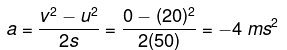(ii) F = ma = 1,000 × (-4) = -4,000 N
(iii) Due to force of friction, the actual force applied by brakes may be slightly less than calculated one.

Ex.9 Which would require greater force: accelerating a 10 g mass at 5 m s_2 or 20 g mass at 2 m s-2
Sol. In first case m1 = 10 g = kg = 0.010 kg;
Now a1 = 5 ms-2 ; F1 = ?
F1 = m1a1 = 0.010 × 5
F1 = 0.050 newton
In second case, m2 = 20 g =0.020 kg
or m2 = 0.020 kg
a2 = 2 m s-2 ; F2 = ?
Now F2 = m2a2 = 0.020 × 2
or F2 = 0.04 newton
We find that F1 > F2, hence more force is required to accelerate 10 g at 5 m s-2 than accelerating 20 g at 2 ms-2.

Ex.10 A truck starts from rest and rolls down a hill with constant acceleration. It travels a distance of 400 m in 20 s. Find its acceleration. Find the force acting on it if its mass is 7 metric ton.
Sol. Here u = 0 m s-1 (Starting from rest); s = 400 m; t = 20 s; a = ?
Using s = ut+1/2 at2,
We have 400 = 0 × a × 20 × 20 = 200 a
or a = 2 ms-2
Now mass m = 7 metric ton = 7,000 kg; F = ?
F = m a = 7,000 × 2
or F = 14,000 N.

Ex.11 A force of 5 N gives a mass m1, an acceleration of 8 ms-2 and a mass m2, an acceleration of 24 m s-2. What acceleration would it give if both the masses are tied together?
Sol. Let us first find mass m1 and m2.
F = m1 a1
5 =m1 (8) or m1 = 5/8 kg
F = m2 a2
5 = m2 (24) or m2 = 5/24 kg
Total mass M = m+ m2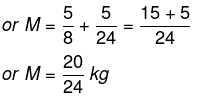Let A be the acceleration produced in mass M.
F = MA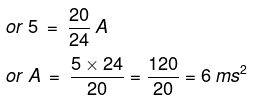Hence the acceleration of the combination is 6 ms-2

Ex.12 A car of mass 1,000 kg moving with a velocity of 40 km h_1 collides with a tree and comes to stop in 5 s. What will be the force exerted by car on the tree?
Sol. Here m = 1,000 kg
u = 40 × ms-1 = ms-1
v = 0 ; t = 5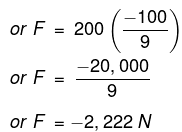Ex.13 The velocity-time graph of a coin moving on a floor is as given in Fig. How much force does the floor exert on the coin? Given the mass of the coin is 10 g.
Sol. Here, u = 24 cm s-1
v = 0 ms-1; t = 8 s ; a = ?
v = u at
F = m a;
Here m = 10 g
F = 10 (-3) = - 30 dyne = - 30/(105) N
or F = -3 × 10-4 N.
Negative sign tells us that the force is acting in the direction opposite to the direction of motion.

Ex.14 An iron sphere of mass 10 kg is dropped from a height of 80 cm. If the downward acceleration of the ball is 10 ms-2, calculate the momentum transferred to the ground by the ball.
Sol. Here, Initial velocity of sphere, u = 0
Distance travelled, s = 80 cm = 0.8 m
Acceleration of sphere, a = 10 ms-2

Step 1. Final velocity of sphere when it just reaches the ground can be calculated using
v2 - u2 = 2as
v2 - 0 = 2 × 10 ms-2 × 0.8 m = 16 m2s-2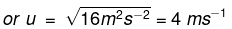Momentum of the sphere just before it touches the ground = mu = 10 kg × 4 ms1 = 40 kg ms-1

Step 2. On reaching the ground, the iron sphere comes to rest, so its final momentum = 0
According to the law of conservation of momentum,
Momentum transferred to the ground = momentum of the sphere just before it comes to rest = 40 kg ms-1.

Ex.15 Two bodies each of mass 0.5 kg are moving in a straight line but opposite in direction with the same velocity of 2 ms1. They collide with each other and stick to each other after collision. What is the common velocity of these bodies after collision?
Sol. Let one body is moving towards left side and and second body is moving to the right side. So velocity of the body to the left side is taken as negative and velocity of body moving to the right side is taken as positive.
Momentum of body moving to left side before collision = mu = 0.5 kg × (2 ms-1) = 1 kg ms-1.

The document Numerical Problems(Solved): Force and Newton's Law of Motion | Science Class 9 is a part of the Class 9 Course Science Class 9.
All you need of Class 9 at this link: Class 9

## Science Class 9

66 videos|355 docs|97 tests

## FAQs on Numerical Problems(Solved): Force and Newton's Law of Motion - Science Class 9

 1. What is the relation between force and Newton's Law of Motion?Ans. According to Newton's Law of Motion, the force acting on an object is directly proportional to its mass and acceleration. This means that if the force acting on an object increases, its acceleration will also increase proportionally.
 2. How do you calculate force?Ans. The formula to calculate force is F = m x a, where F is the force, m is the mass of the object, and a is the acceleration.
 3. What are the three laws of motion given by Newton?Ans. Newton's three laws of motion are: 1. An object at rest will remain at rest, and an object in motion will remain in motion unless acted upon by an external force. 2. The force acting on an object is directly proportional to its mass and acceleration. 3. For every action, there is an equal and opposite reaction.
 4. How does Newton's third law of motion apply to real-life situations?Ans. Newton's third law of motion states that for every action, there is an equal and opposite reaction. This means that when an object exerts a force on another object, the second object exerts an equal and opposite force on the first object. For example, when you jump off a diving board, your feet push down on the board, and the board pushes up on your feet with an equal and opposite force, propelling you upwards.
 5. What is the difference between mass and weight?Ans. Mass is the amount of matter present in an object, while weight is the force exerted by gravity on the object. Mass is measured in kilograms, while weight is measured in newtons. The weight of an object can vary depending on the gravitational force acting on it, but its mass remains the same regardless of the gravitational force.

## Science Class 9

66 videos|355 docs|97 testsExplore Courses for Class 9 examSignup to see your scores go up within 7 days! Learn & Practice with 1000+ FREE Notes, Videos & Tests.
10M+ students study on EduRev
Track your progress, build streaks, highlight & save important lessons and more!
Related Searches

,

,

,

,

,

,

,

,

,

,

,

,

,

,

,

,

,

,

,

,

,

;